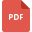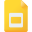# multiplication tutorialsTwo-Step Multiplication WorksheetMultiplying Two-Digit Numbers WorksheetEquivalent Fractions by Multiplication and Division
Fraction, Grade 6, United States, MathematicsProperties of Multiplication
Multiplication, Grade 5, United States, MathematicsAddition and Multiplication Properties for Real NumbersHow to Multiply a Number by a Two-Digit NumberMulitplying by Whole Number
Mathematics, Grade 7, United States, MultiplicationSimplifying Algebraic Expressions Multiplying and Dividing
Multiplication, Grade 8, United States, DivisionMultiplying by 10, 100, and 1,000
Multiplication, Grade 5, United States, MathematicsDirected numbers: Multiplication and Division of Integers
Multiplication, Grade 8, United States, Integers, Division, MathematicsMultiplication and Division Algorithms
Mathematics, Grade 7, United States, Multiplication, DivisionCommutative and Associative Property of Multiplication
Properties of Multiplication, Grade 3, United StatesMultiplying Fractions and Mixed Numbers
Fraction, Grade 5, United States, MultiplicationSolving Basic Equations with Multiplication or Division
Multiplication, Grade 8, United States, DivisionMultiplication and Division Word/Story Problems
Multiplication, Grade 4, United States, Money Word Problems, Mathematics, DivisionMultiplying Mixed Numbers (Mixed Numerals)
Multiplication, Grade 8, United States, MathematicsVisual Models of Multiplication and Division
Mathematics, Grade 3, United States, Multiplication, DivisionAddition, Subtraction, Multiplication, Division of Fractions
Fraction, Grade 5, United States, MathematicsMultiply Multi-Digit by 1-Digit Number
Multiplication, Grade 3, United States, MathematicsMultiplying and Dividing an Algebraic Expression
Mathematics, Grade 7, United States, Multiplication, DivisionCommutative Property of Multiplication
Multiplication, Grade 3, United States, Number SystemDetailed Explanation of Estimating Products
Multiplication, Grade 5, United States, MathematicsA Parent's Guide to solve Multiplication and Division Word Problems
Multiplication, Grade 3, United States, Number SystemMultiplication StrategiesSolving Equations containing Multiplication and Division
Multiplication, Grade 8, United States, DivisionDecimals and Division, Grade 6, United States, Decimals and MultiplicationLearn to Model Multiplication
Multiplication, Grade 3, United States, Number SystemMultiplication with the Vedic Method
Vedic Mathematics, Class 9, IndiaEqual Groups Multiplication Worksheet
Multiplication, Grade 3, United States, CountingExpanding Algebraic Expressions: Multiplication
Multiplication, Grade 8, United States, MathematicsMultiplication of Decimals by Decimals to Two Decimal Places Worksheet
Decimals and Multiplication, Grade 8, United States, MathematicsMultiplication and Division of Fractions
Fraction, Grade 5, United States, MultiplicationDirected Numbers: Multiplication and Division Worksheet
Multiplication, Grade 8, United States, DivisionMultiplying Fractions Worksheet
Fraction, Grade 8, United States, MultiplicationAddition and Multiplication Properties for Real Numbers